﻿ 损伤悬臂梁固有频率精确测量与分析 Measurement and Analysis of Natural Frequency of Damaged Cantilever Beam

Open Journal of Acoustics and Vibration
Vol. 07  No. 01 ( 2019 ), Article ID: 29153 , 8 pages
10.12677/OJAV.2019.71003

Measurement and Analysis of Natural Frequency of Damaged Cantilever Beam

Hanqing Li1, Jinwu Wu1*, Xiaojun Guo2, Wenhao Tian1, Wei Li1, Liang Chen1

1College of Aircraft Engineering, Nanchang Hangkong University, Nanchang Jiangxi

2Zhuzhou Aviation Powerplant Research Institute, Zhuzhou Hunan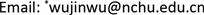Received: Feb. 14th, 2019; accepted: Feb. 28th, 2019; published: Mar. 7th, 2019ABSTRACT

The relationship between the damage position of cantilever beam and the natural frequency change before and after structural damage is derived. In order to reduce the influence of the clamping force fluctuation of the fixed end of the cantilever beam on the measurement results, a set of natural frequency measurement method with high precision is designed. The accuracy of the method is verified by experiments. It is proved that the average relative error of the measured natural frequencies can be reduced to less than 0.7%. It calculated and analyzed the natural frequency of the cantilever beam before and after the damage. The results show that the damage position of the structure obtained by the natural frequency change is basically consistent with the actual position.

Keywords:Cantilever Beam Structure, Natural Frequency, Vibration Measurement, Damage Detection

1南昌航空大学飞行器工程学院，江西 南昌

2株洲航空动力机械研究所，湖南 株洲Copyright © 2019 by author(s) and Hans Publishers Inc.

This work is licensed under the Creative Commons Attribution International License (CC BY).

http://creativecommons.org/licenses/by/4.0/1. 引言

2. 理论分析

$\left(K-{\omega }^{2}M\right)\phi =0$ (1)

$\left[\left(K+\Delta K\right)-\left({\omega }^{2}+\Delta {\omega }^{2}\right)\left(M+\Delta M\right)\right]\left(\phi +\Delta \phi \right)=0$ (2)

$\Delta {\omega }^{2}=\frac{{\phi }^{\text{T}}\Delta K\phi }{{\phi }^{\text{T}}M\phi }$ (3)

$\Delta {\omega }^{2}=\frac{{\phi }_{i}^{\text{T}}\Delta K{\phi }_{i}}{{\phi }_{i}^{\text{T}}M{\phi }_{i}}$ (4)

$\Delta {\omega }_{i}^{2}=\frac{\underset{n}{\sum }{\epsilon }_{n}^{\text{T}}\left({\phi }_{i}\right)\Delta {K}_{n}{\epsilon }_{n}\left({\phi }_{i}\right)}{{\phi }_{i}^{\text{T}}M{\phi }_{i}}$ (5)

$\Delta {\omega }_{i}^{2}=\frac{{\epsilon }_{n}^{\text{T}}\left({\phi }_{i}\right)\Delta {K}_{n}{\epsilon }_{n}\left({\phi }_{i}\right)}{{\phi }_{i}^{\text{T}}M{\phi }_{i}}$ (6)

$\Delta {\omega }_{i}^{2}={\lambda }_{i}\left({\alpha }_{n},n\right)$ (7)

${\alpha }_{n}=\frac{\Delta {K}_{n}}{{K}_{n}}$ (8)

$\Delta {\omega }_{i}^{2}=\frac{{\alpha }_{n}{\epsilon }_{n}^{\text{T}}\left({\phi }_{i}\right){K}_{n}{\epsilon }_{n}\left({\phi }_{i}\right)}{{\phi }_{i}^{\text{T}}M{\phi }_{i}}$ (9)

 (10)

$B={\underset{i}{\sum }\left[{\left(|\frac{\Delta {\omega }_{i}}{\Delta {\omega }_{1}}|\right)}_{O}-{\left(|\frac{\Delta {\omega }_{i}}{\Delta {\omega }_{1}}|\right)}_{C}\right]}^{2}$ (11)

3. 测量方法及装置

3.1. 原理及测量说明

3.1.1. 原理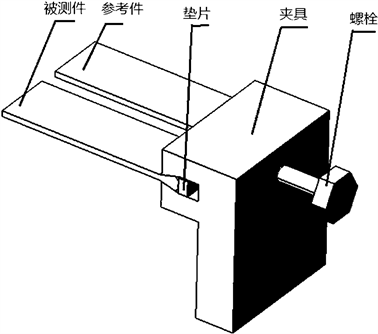Figure 1. Measuring device and device assembly

3.1.2. 测量说明

3.2. 实验验证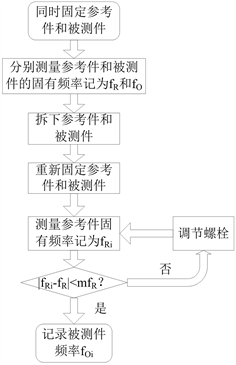Figure 2. Measurement process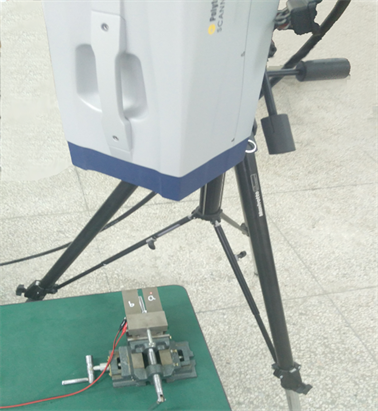Figure 3. Experimental measurementTable 1. Measurement data of the test piece and reference piece without damage

4. 利用固有频率变化对损伤悬臂梁分析

4.1. 对悬臂梁损伤处理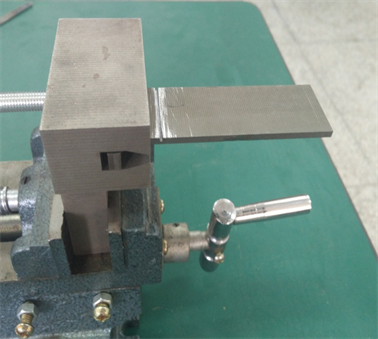Figure 4. Damaged cantilever beam

4.2. 分析损伤悬臂梁的固有频率变化

4.2.1. 实验测量悬臂梁损伤前后频率变化Table 2. Natural frequency of damaged cantilever beam

4.2.2. 有限元分析悬臂梁频率变化Table 3. Natural frequency calculation of cracks at different positions

5. 结论

1) 详细推导了悬臂梁损伤定位公式，得出了结构损伤前后固有频率变化的比值仅是损伤位置的函数。采用该方法可估算出只有单一损伤时悬臂梁损伤位置。

2) 制定了一套能够提高悬臂梁固有频率测量精度的方法。在实际工程应用中可减小由约束条件波动对测量结果造成的影响。对于文章所用试件，可将测量的平均相对误差控制在0.7%以下。

3) 制作了简化的航空发动机叶片模型。采用文章所述的测量方法和损伤定位公式，得到的损伤位置计算值与实际值一致。验证了损伤定位公式和固有频率测量方法的有效性。利用固有频率的变化计算结构的损伤位置具有定位简便，精度高，成本低的优点。

Measurement and Analysis of Natural Frequency of Damaged Cantilever Beam[J]. 声学与振动, 2019, 07(01): 20-27. https://doi.org/10.12677/OJAV.2019.71003

1. 1. 马楠楠, 陶春虎, 何玉怀. 航空发动机叶片多轴疲劳试验研究进展[J]. 航空材料学报, 2012, 32(6): 44-49.

2. 2. 于霞. 飞机发动机叶片缺陷的电磁检测技术研究[D]: [博士学位论文]. 北京: 北京理工大学, 2014.

3. 3. 尤敬业, 何娟娟. 航空发动机叶片检测与裂纹分析[J]. 计测技术, 2016, 36(S1): 74-75.

4. 4. 洪杰, 刘书国, 张大义, 陈萌. 小型短寿命涡扇发动机涡轮叶片疲劳失效分析[J]. 航空动力学报, 2012, 27(3): 604-609.

5. 5. 郭隆清. 基于固有频率的悬臂梁裂纹参数识别方法研究[D]: [硕士学位论文]. 南昌: 南昌航空大学, 2016.

6. 6. 黄红蓝, 赵永信, 梁巍, 等. 高阶固有频率检测评估旧零件疲劳损伤的研究[J]. 广西大学学报(自然科学版), 2017, 42(3): 990-1000.

7. 7. 王乐, 杨智春, 谭光辉, 等. 基于固有频率向量的结构损伤检测方法[J]. 应用力学学报, 2008, 25(4): 709-713, 742.

8. 8. 韩大建, 王文东. 基于振动的结构损伤识别方法的近期研究进展[J]. 华南理工大学学报(自然科学版), 2003(1): 91-96.

9. 9. Yang, Q.W. and Liu, J.K. (2006) A Coupled Method for Structural Damage Identification. Journal of Sound and Vibration, 296, 401-405. https://doi.org/10.1016/j.jsv.2006.02.014

10. 10. Khiem, N.T. and Toan, L.K. (2014) A Novel Method for Crack Detection in Beam-Like Structures by Measurements of Natural Frequencies. Journal of Sound and Vibration, 333, 4084-4103. https://doi.org/10.1016/j.jsv.2014.04.031